# Difference between Convert.ToInt32 vs Int32.Parse

Wikitechy | 5716 Views | c# | 14 Jun 2016

#### Syntax:

`Int32.Parse (string s)`
• Int32.Parse (string s) method converts the string representation of a number to its 32-bit signed integer equivalent.
• When “s” is a null reference, it will throw ArgumentNullException.
• If “s” is other than integer value, it will throw FormatException.
• When “s” represents a number less than MinValue or greater than MaxValue, it will throw OverflowException.

#### For example:

`using System.Collections.Generic;using System.Linq;using System.Text;namespace Convert.ToInt32_vs_Int32.Parse{    class Program    {        static void Main(string[] args)        {            string val1 = "7777";            string val2 = "777.77";            string val3 = null;            string val4 = "77777777777777777777123456789";            int result;            result = Int32.Parse(val1);            ///An unhandled exception of type 'System.FormatException'             ///occurred in mscorlib.dll            result = Int32.Parse(val2);                        //An unhandled exception of type 'System.ArgumentNullException'            //occurred in mscorlib.dll            result = Int32.Parse(val3);            //An unhandled exception of type 'System.OverflowException'             ///occurred in mscorlib.dll            result = Int32.Parse(val4);         }    }}`

#### Code Explanation: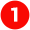Here we define the library files.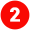In this line we define the namespace “Convert.ToInt32_vs_Int32.Parse”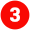In this program the class name as “program” is defined.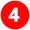public static void main(String[] args)

• public is the visibility. This can be public, private, protected or default.
• static is a special [optional] keyword that indicates that this method can be called without creating an instance of this class.
• void is the return type of this method, indicating that this method doesn't return anything.
• main( ... ) is the name of this method. The parentheses indicate that this is a method.
• String[] args is a single parameter for the method. String[] is the type of the parameter, indicating an array of Strings. args is the name of the parameter.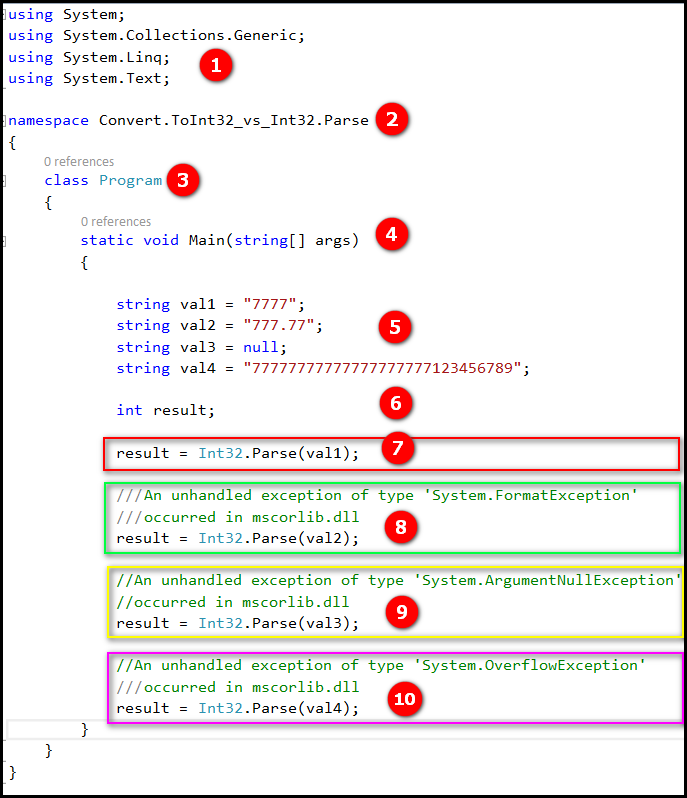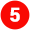In this section we define the string variable var1, var2, var3, var4 and their values.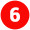In this line we declare the variable “result” as integer.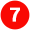Here in this line the variable “var1” value will be parsed using the method Int32.Parse() returns the value “77777” which will be stored in the variable “result”.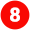Similarly, variable “var2” value will be parsed using the method Int32.Parse() and returns 'System.FormatException' which will be stored in the variable “result”.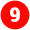Same as that variable “var3” value will be parsed using the method Int32.Parse() returns 'System.ArgumentNullException' which will be stored in the variable “result”.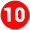In this line,the variable “var4” value will be parsed using the method Int32.Parse() returns 'System.OverflowException' which will be stored in the variable “result”.

#### Syntax:

`Convert.ToInt32(string s)`
• Convert.ToInt32(string s) method converts the specified string representation of 32-bit signed integer equivalent. This calls in turn Int32.Parse () method.
• “s” is a null reference, it will return “0” rather than throwing an ArgumentNullException.
• If “s” is other than integer value, it will throw FormatException.
• When “s” represents a number less than MinValue or greater than MaxValue, it will throw OverflowException.

#### For example:

`using System;using System.Collections.Generic;using System.Linq;using System.Text;namespace Convert.ToInt32_vs_Int32.Parse{    class Program    {        static void Main(string[] args)        {            string val1 = "7777";            string val2 = "777.77";            string val3 = null;            string val4 = "77777777777777777777123456789";            int result;            //result 7777            result = System.Convert.ToInt32(val1);            ///An unhandled exception of type 'System.FormatException'             ///occurred in mscorlib.dll            result = System.Convert.ToInt32(val2);                        //value is 0            result = System.Convert.ToInt32(val3);            //An unhandled exception of type 'System.OverflowException'             ///occurred in mscorlib.dll            result = System.Convert.ToInt32(val4);         }    }}`

#### Code Explanation: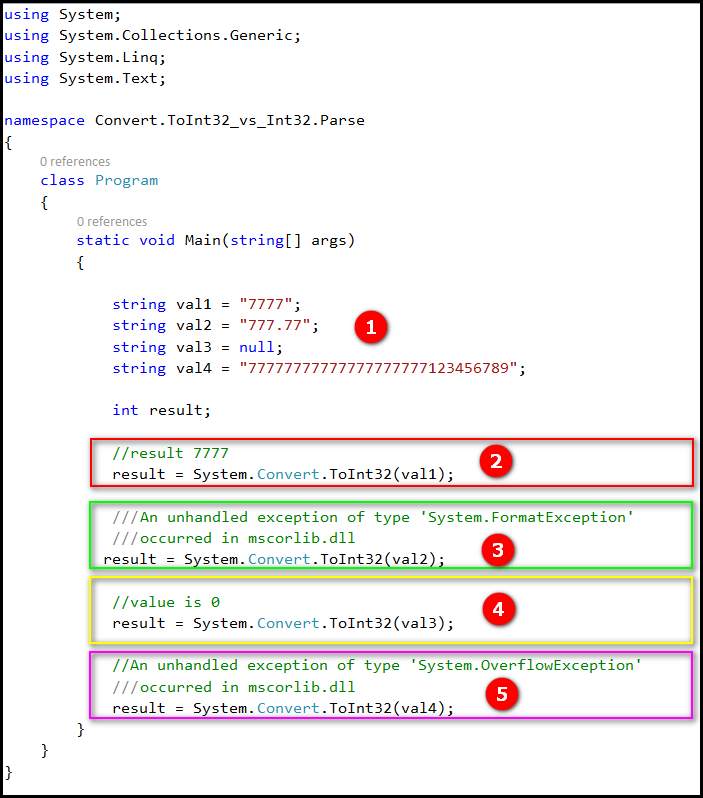In this section we define the string variable var1, var2, var3, var4 and their values.Here in this line the variable “var1” value will be parsed using the method System.Convert.ToInt32() that returns the value “77777” which will be stored in the variable “result”.Similarly, variable “var2” value will be parsed using the method System.Convert.ToInt32() that  returns 'System.FormatException' which will be stored in the variable “result”.Same as that variable “var3” value will be parsed using the method System.Convert.ToInt32() that returns “0”  which will be stored in the variable “result”.In this line variable “var4” value will be parsed using the method System.Convert.ToInt32() that returns 'System.OverflowException' which will be stored in the variable “result”.

#### Applies to:

• Visual Studio 2008
• Visual Studio 2010
• Visual Studio 2012
• Visual Studio 2013
• Visual Studio 2015
• Visual Studio "15"

#### Related Tags:

• Difference between int.Parse,Convert.ToInt32 and int.TryParse
• Difference Between Int32.Parse(), Convert.ToInt32()
• c# - Whats the main difference between int.Parse() and Convert .ToInt32(string)
• c# - What's the difference between Convert.ToInt32 and Int32.Parse()
• c# - Better use int.Parse or Convert.ToInt32
• parsing - Difference between Convert and Parse c#
• What's the difference between (int), Int32.Parse and Convert.ToInt32()
• Difference between int.Parse and Convert.ToInt32
• what is the different between Parse and Convert
• Difference between int.Parse() and Convert.ToInt32

Bug Bounty
Webinar